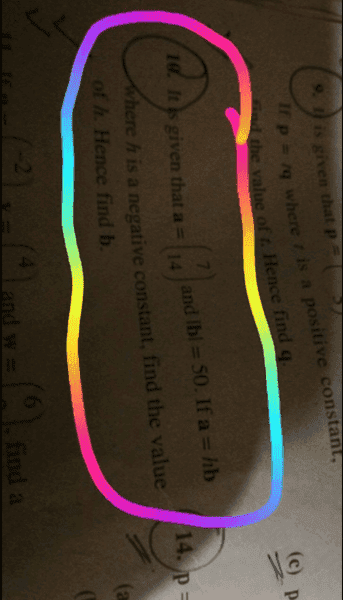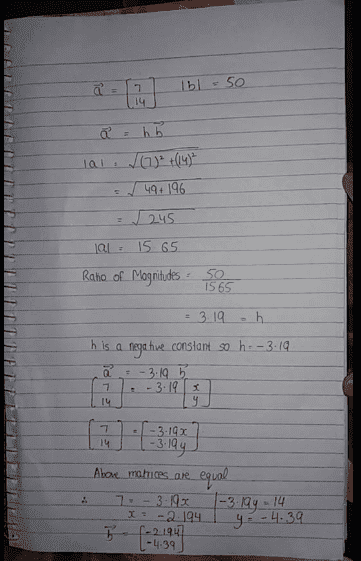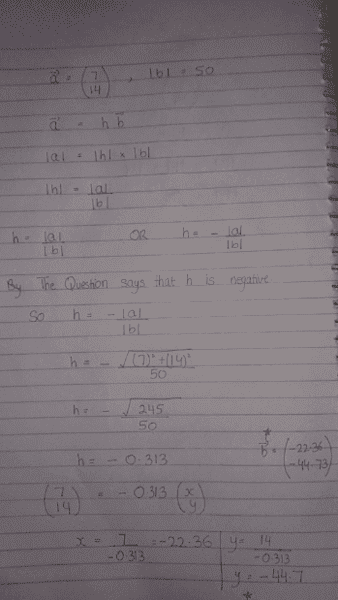# Vectors (Multiplication)

Member warned to take more care when posting images

## Homework Statement## Homework Equations

Is my solution correct? If not then please point out the mistakes and help me solve this question in the right way. Thanks in advance.

## The Attempt at a Solution#### Attachments

.Scott
Homework Helper
|a| = |b| |h|
so
|h| = |a| / |b|
and h<0

•|a| = |b| |h|
so
|h| = |a| / |b|
and h<0
After finding h, can we solve this question by matrix method? (The way I did)

.Scott
Homework Helper
You have h = -|b|/|a|.
It should be h = -|a|/|b|.

Then solve with b = a/h

•You have h = -|b|/|a|.
It should be h = -|a|/|b|.

Then solve with b = a/h
If we make |h| the subject, it will be |h|= |a| / |b|. The answer will be positive. So | | signs will reverse the signs and give a negative value of h?

.Scott
Homework Helper
Right. We need to make h negative.
|a|/|b| is positive over positive, which is positive.

Per the problem, we need h to be negative...
So, h = -|a|/|b|

•Right. We need to make h negative.
|a|/|b| is positive over positive, which is positive.

Per the problem, we need h to be negative...
So, h = -|a|/|b|
How will it become negative?

If I use the equation |a| = |h| |b| then how can I get a negative value of h?

.Scott
Homework Helper
How will it become negative?

If I use the equation |a| = |h| |b| then how can I get a negative value of h?

By using the equation: h = -|a|/|b|

Here's the sequence:
|a| = |h| |b|
|a|/|b| = |h|
so either h = |a|/|b|
or h = -|a|/|b|

But we know from the problem statement that it must be negative.

•By using the equation: h = -|a|/|b|
By removing ''| |'' from h, the other side will become negative?

.Scott
Homework Helper
By removing ''| |'' from h, the other side will become negative?
I added some text to my last post.
Here it is again:

Here's the whole sequence:
|a| = |h| |b|
|a|/|b| = |h|
so either h = |a|/|b|
or h = -|a|/|b|

But we know from the problem statement that it must be negative.

•I added some text to my last post.
Here it is again:

Here's the whole sequence:
|a| = |h| |b|
|a|/|b| = |h|
so either h = |a|/|b|
or h = -|a|/|b|

But we know from the problem statement that it must be negative.
Like this?#### Attachments

.Scott
Homework Helper
Yes!!

•Yes!!
Thank you!!!!

Ray Vickson
Homework Helper
Dearly Missed

## Homework Statement

View attachment 227388

## Homework Equations

Is my solution correct? If not then please point out the mistakes and help me solve this question in the right way. Thanks in advance.

## The Attempt at a Solution

View attachment 227389

Your problem is totally unreadable. Please take the trouble to adhere to the PF standard, by typing out the statement of the problem. If you cannot do that, you should at least make sure you take a proper photo that will come out readable by the rest of the world.